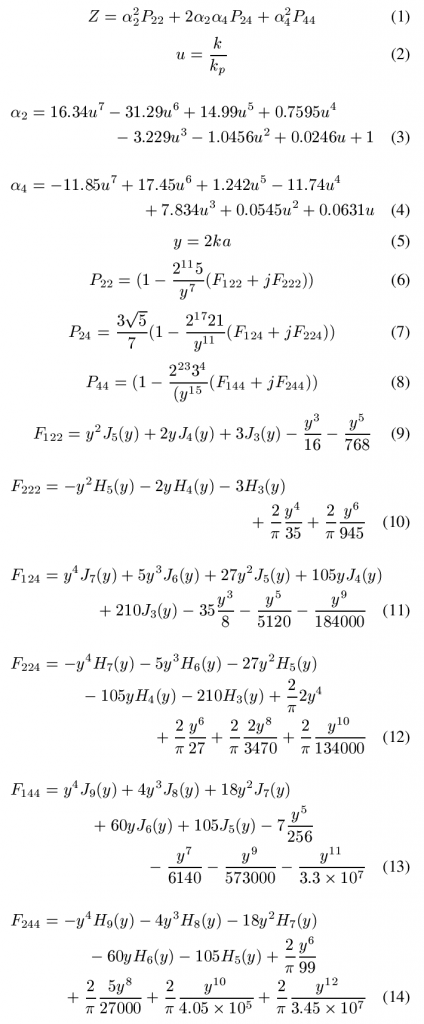The radiation impedance seen by a single circular CMUT cell in the absence of any neighboring cells is shown in figure as a function of ka, where k is the wavenumber in the immersion medium and a is the radius of the top plate. The radiation impedance shown is normalized with respect to 0c0 where S is the area of the top plate of the CMUT cell, ρ0 is the density and c0  is the sound velocity of the immersion medium.

In the equations below

• Z : normalized complex radiation impedance
• k : wavenumber in the immersion medium
• kp : wavenumber of the second order circularly symmetric mode of the CMUT top plate
• a : radius of the CMUT top plateMutual Impedance between two neighboring CMUT cells

The mutual impedance (normalized with respect to 0c0) between two circular CMUT cells with a center-to-center distance of d:

Z=(sin kd +j cos kd)/(kd)